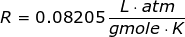## Thursday, April 30, 2015

### Dalton's Law of Partial Pressure

Category: Chemical Engineering Math

"Published in Newark, California, USA"

How many grams of KClO3 are needed to prepare 1.8 L of O2 gas that is collected over H2O at 22°C and 760 torr? Vapor pressure of water at 22°C is 19.8 torr.

Solution:

From the given word problem, it is about Dalton's Law of Partial Pressure because it involves the mixture of gas vapors. In this problem, oxygen gas that is collected from the decomposition of KClO3 over water is not a pure oxygen. It is a mixture of pure oxygen and water vapor. By Dalton's Law of Partial Pressure, the pressure of pure oxygen is

The absolute temperature of oxygen gas is

The universal gas law constant for gmole, K, and mm Hg (torr) is.

By Ideal Gas Law, we can calculate the number of moles of oxygen gas as follows

From the decomposition of KClO3 by heating,

Therefore, the amount of KClO3 is

## Wednesday, April 29, 2015

### Ideal Gas Law Problems

Category: Chemical Engineering Math

"Published in Newark, California, USA"

What is the volume of 18.0 grams of pure water at 4°C and 1 atm?

Solution:

From the given word problem, it is about Ideal Gas Law because the weight, temperature, and pressure of pure water are given and we need to solve for the volume.

The Ideal Gas Law is given by the equation

where:

P = pressure of a gas or vapor
V =  volume of a gas or vapor
n = moles of a gas or vapor
T = absolute temperature of a gas or vapor
n = universal gas law constant

The number of moles of pure water is

The absolute temperature of pure water is

The universal gas law constant for gmole, K, and atmosphere is.

Therefore, the volume of pure water is